# Data and Model Objects in System Identification Toolbox™

This example shows how to manage data and model objects available in the System Identification Toolbox™. System identification is about building models from data. A data set is characterized by several pieces of information: The input and output signals, the sample time, the variable names and units, etc. Similarly, the estimated models contain information of different kinds - estimated parameters, their covariance matrices, model structure and so on.

This means that it is suitable and desirable to package relevant information around data and models into objects. System Identification Toolbox™ contains a number of such objects, and the basic features of these are described in this example.

### The IDDATA Object

First create some data:

```u = sign(randn(200,2)); % 2 inputs y = randn(200,1); % 1 output ts = 0.1; % The sample time ```

To collect the input and the output in one object do

```z = iddata(y,u,ts); ```

The data information is displayed by just typing its name:

```z ```
```z = Time domain data set with 200 samples. Sample time: 0.1 seconds Outputs Unit (if specified) y1 Inputs Unit (if specified) u1 u2 ```

The data is plotted as iddata by the `plot` command, as in `plot(z)`. Press a key to continue and advance between the subplots. Here, we plot the channels separately:

```plot(z(:,1,1)) % Data subset with Input 1 and Output 1. ```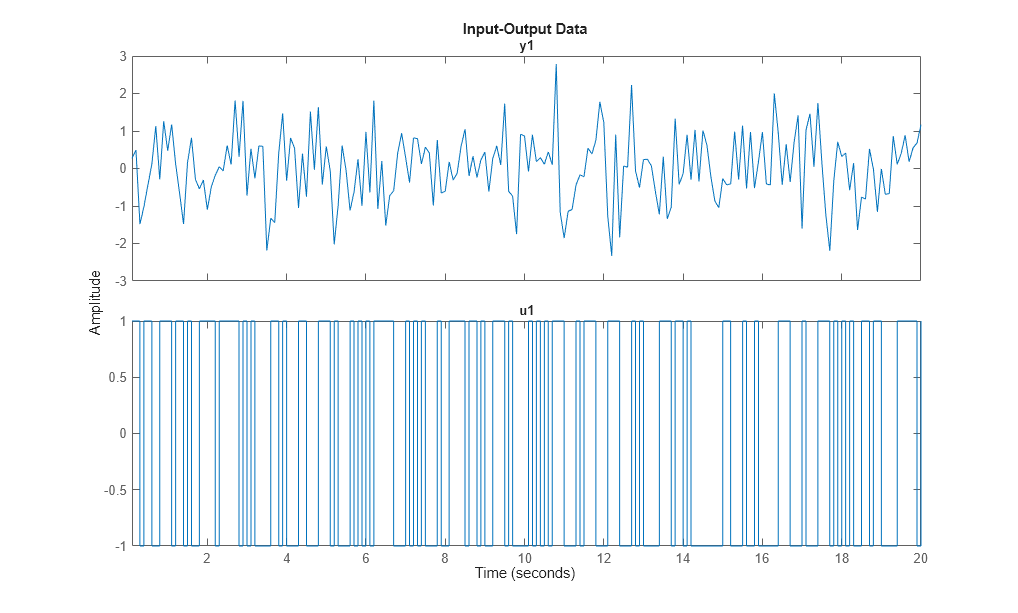```plot(z(:,1,2)) % Data subset with Input 2 and Output 1. ```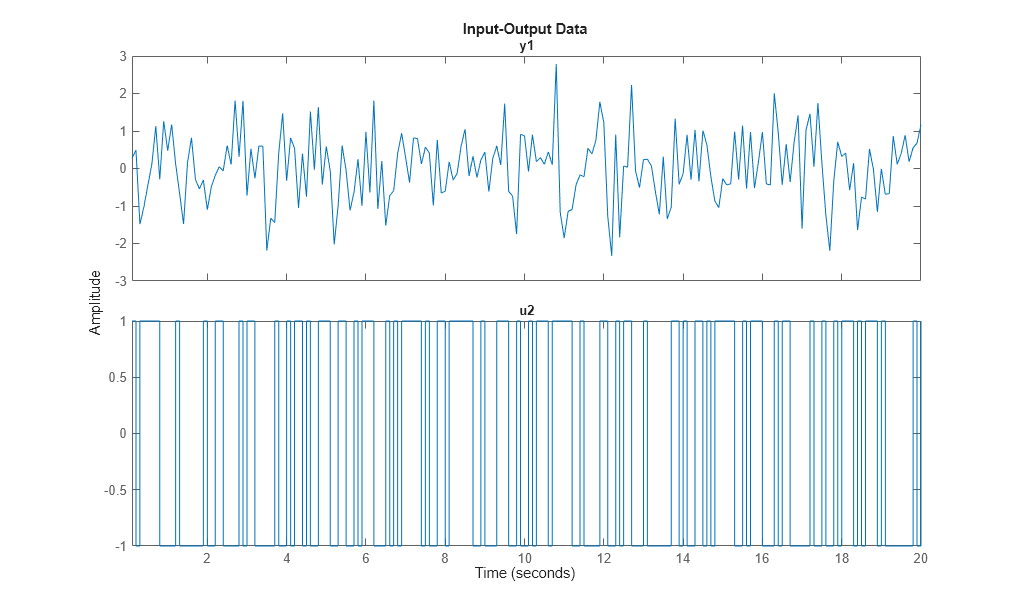To retrieve the outputs and inputs, use

```u = z.u; % or, equivalently u = get(z,'u'); y = z.y; % or, equivalently y = get(z,'y'); ```

To select a portion of the data:

```zp = z(48:79); ```

To select the first output and the second input:

```zs = z(:,1,2); % The ':' refers to all the data time points. ```

The sub-selections can be combined:

```plot(z(45:54,1,2)) % samples 45 to 54 of response from second input to the first output. ```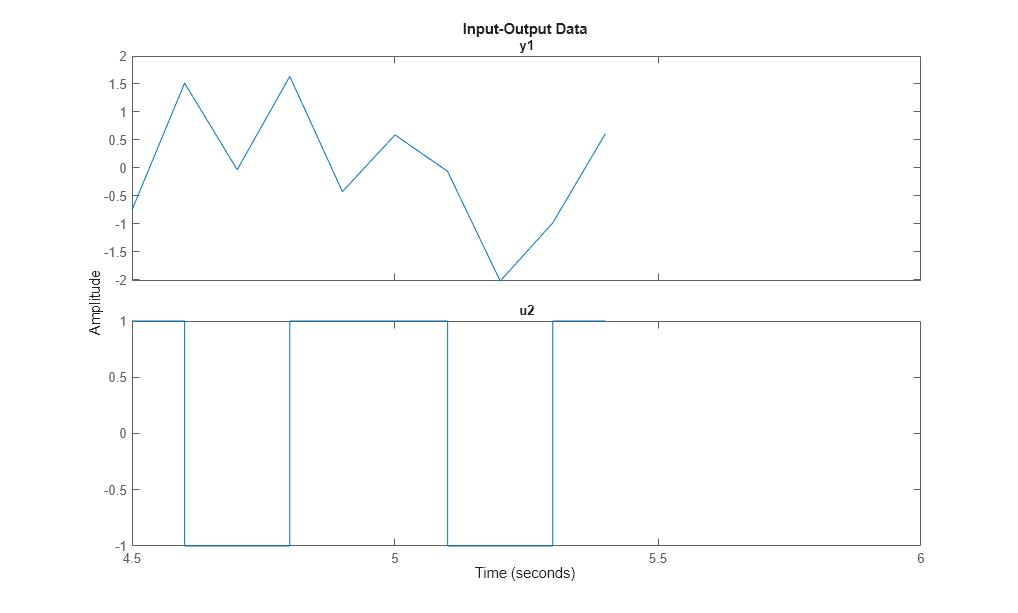The channels are given default names 'y1', 'u2', etc. This can be changed to any values by

```set(z,'InputName',{'Voltage';'Current'},'OutputName','Speed'); ```

Equivalently we could write

```z.inputn = {'Voltage';'Current'}; % Autofill is used for properties z.outputn = 'Speed'; % Upper and lower cases are also ignored ```

For bookkeeping and plots, also units can be set:

```z.InputUnit = {'Volt';'Ampere'}; z.OutputUnit = 'm/s'; z ```
```z = Time domain data set with 200 samples. Sample time: 0.1 seconds Outputs Unit (if specified) Speed m/s Inputs Unit (if specified) Voltage Volt Current Ampere ```

All current properties are (as for any object) obtained by get:

```get(z) ```
```ans = struct with fields: Domain: 'Time' Name: '' OutputData: [200x1 double] y: 'Same as OutputData' OutputName: {'Speed'} OutputUnit: {'m/s'} InputData: [200x2 double] u: 'Same as InputData' InputName: {2x1 cell} InputUnit: {2x1 cell} Period: [2x1 double] InterSample: {2x1 cell} Ts: 0.1000 Tstart: [] SamplingInstants: [200x0 double] TimeUnit: 'seconds' ExperimentName: 'Exp1' Notes: {} UserData: [] ```

In addition to the properties discussed so far, we have 'Period' which denotes the period of the input if periodic Period = inf means a non-periodic input:

```z.Period ```
```ans = Inf Inf ```

The intersample behavior of the input may be given as 'zoh' (zero-order-hold, i.e. piecewise constant) or 'foh' (first- order-hold, i.e., piecewise linear). The identification routines use this information to adjust the algorithms.

```z.InterSample ```
```ans = 2x1 cell array {'zoh'} {'zoh'} ```

You can add channels (both input and output) by "horizontal concatenation", i.e. z = [z1 z2]:

```z2 = iddata(rand(200,1),ones(200,1),0.1,'OutputName','New Output',... 'InputName','New Input'); z3 = [z,z2] ```
```z3 = Time domain data set with 200 samples. Sample time: 0.1 seconds Outputs Unit (if specified) Speed m/s New Output Inputs Unit (if specified) Voltage Volt Current Ampere New Input ```

Let us plot some of the channels of `z3`:

```plot(z3(:,1,1)) % Data subset with Input 2 and Output 1. ```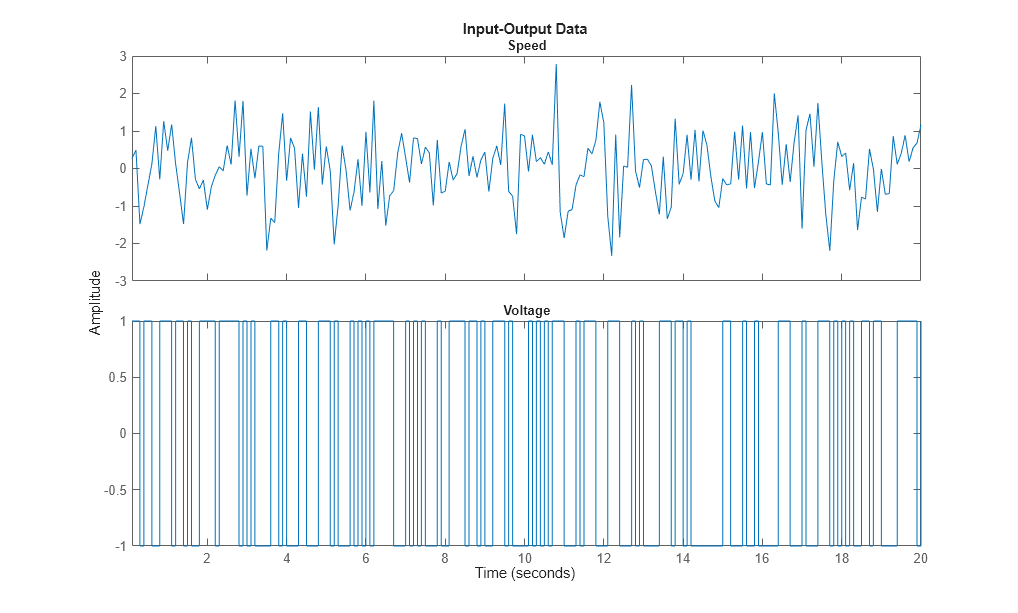```plot(z3(:,2,3)) % Data subset with Input 2 and Output 3. ```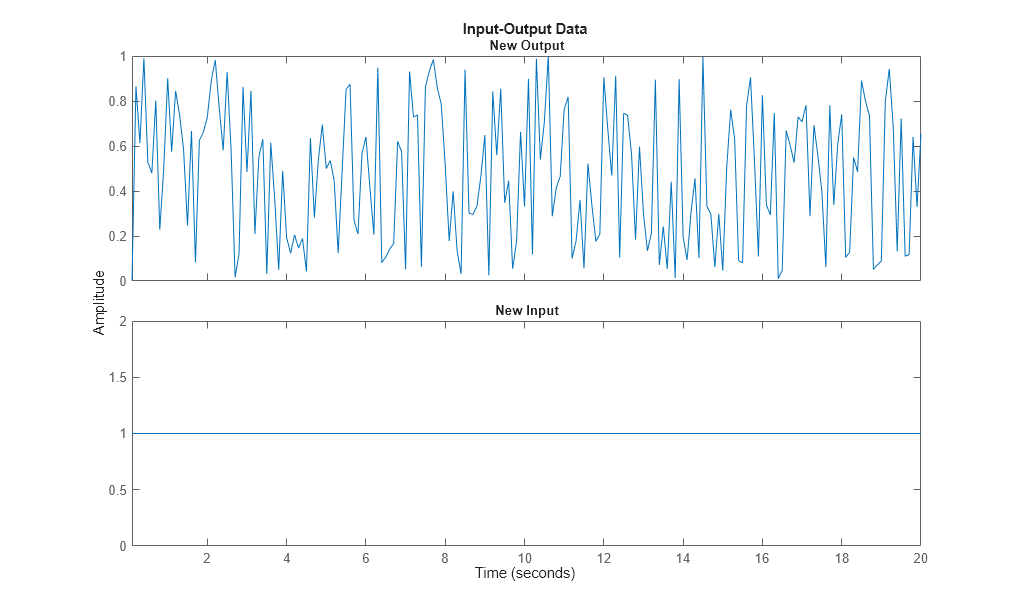Generating Inputs

The command `idinput` generates typical input signals.

```u = idinput([30 1 10],'sine'); % 10 periods of 30 samples u = iddata([],u,1,'Period',30) % Making the input an IDDATA object. ```
```u = Time domain data set with 300 samples. Sample time: 1 seconds Inputs Unit (if specified) u1 ```

SIM applied to an iddata input delivers an iddata output. Let us use `sim` to obtain the response of an estimated model `m` using the input `u`. We also add noise to the model response in accordance with the noise dynamics of the model. We do this by using the "AddNoise" simulation option:

```m = idpoly([1 -1.5 0.7],[0 1 0.5]); % This creates a model; see below. options = simOptions; options.AddNoise = true; y = sim(m,u,options) % simulated response produced as an iddata object ```
```y = Time domain data set with 300 samples. Sample time: 1 seconds Outputs Unit (if specified) y1 ```

The simulation input `u` and the output `y` may be combined into a single `iddata` object as follows:

```z5 = [y u] % The output-input iddata. ```
```z5 = Time domain data set with 300 samples. Sample time: 1 seconds Outputs Unit (if specified) y1 Inputs Unit (if specified) u1 ```

More about the `iddata` object is found under `help iddata`.

### The Linear Model Objects

All models are delivered as MATLAB® objects. There are a few different objects depending on the type of model used, but this is mostly transparent.

```load iddata1 m = armax(z1,[2 2 2 1]); % This creates an ARMAX model, delivered as an IDPOLY object ```

All relevant properties of this model are packaged as one object (here, `idpoly`). To display it just type its name:

```m ```
```m = Discrete-time ARMAX model: A(z)y(t) = B(z)u(t) + C(z)e(t) A(z) = 1 - 1.531 z^-1 + 0.7293 z^-2 B(z) = 0.943 z^-1 + 0.5224 z^-2 C(z) = 1 - 1.059 z^-1 + 0.1968 z^-2 Sample time: 0.1 seconds Parameterization: Polynomial orders: na=2 nb=2 nc=2 nk=1 Number of free coefficients: 6 Use "polydata", "getpvec", "getcov" for parameters and their uncertainties. Status: Estimated using ARMAX on time domain data "z1". Fit to estimation data: 76.38% (prediction focus) FPE: 1.127, MSE: 1.082 ```
` Many of the model properties are directly accessible`
```m.a % The A-polynomial ```
```ans = 1.0000 -1.5312 0.7293 ```

A list of properties is obtained by get:

```get(m) ```
``` A: [1 -1.5312 0.7293] B: [0 0.9430 0.5224] C: [1 -1.0587 0.1968] D: 1 F: 1 IntegrateNoise: 0 Variable: 'z^-1' IODelay: 0 Structure: [1x1 pmodel.polynomial] NoiseVariance: 1.1045 Report: [1x1 idresults.polyest] InputDelay: 0 OutputDelay: 0 Ts: 0.1000 TimeUnit: 'seconds' InputName: {'u1'} InputUnit: {''} InputGroup: [1x1 struct] OutputName: {'y1'} OutputUnit: {''} OutputGroup: [1x1 struct] Notes: [0x1 string] UserData: [] Name: '' SamplingGrid: [1x1 struct] ```

Use `present` to view the estimated parameter covariance as +/- 1 standard deviation uncertainty values on individual parameters:

```present(m) ```
``` m = Discrete-time ARMAX model: A(z)y(t) = B(z)u(t) + C(z)e(t) A(z) = 1 - 1.531 (+/- 0.01801) z^-1 + 0.7293 (+/- 0.01473) z^-2 B(z) = 0.943 (+/- 0.06074) z^-1 + 0.5224 (+/- 0.07818) z^-2 C(z) = 1 - 1.059 (+/- 0.06067) z^-1 + 0.1968 (+/- 0.05957) z^-2 Sample time: 0.1 seconds Parameterization: Polynomial orders: na=2 nb=2 nc=2 nk=1 Number of free coefficients: 6 Use "polydata", "getpvec", "getcov" for parameters and their uncertainties. Status: Termination condition: Near (local) minimum, (norm(g) < tol).. Number of iterations: 3, Number of function evaluations: 7 Estimated using ARMAX on time domain data "z1". Fit to estimation data: 76.38% (prediction focus) FPE: 1.127, MSE: 1.082 More information in model's "Report" property. ```

Use `getpvec` to fetch a flat list of all the model parameters, or just the free ones, and their uncertainties. Use `getcov` to fetch the entire covariance matrix.

```[par, dpar] = getpvec(m, 'free') CovFree = getcov(m,'value') ```
```par = -1.5312 0.7293 0.9430 0.5224 -1.0587 0.1968 dpar = 0.0180 0.0147 0.0607 0.0782 0.0607 0.0596 CovFree = 0.0003 -0.0003 0.0000 0.0007 0.0004 -0.0003 -0.0003 0.0002 -0.0000 -0.0004 -0.0003 0.0002 0.0000 -0.0000 0.0037 -0.0034 -0.0000 0.0001 0.0007 -0.0004 -0.0034 0.0061 0.0008 -0.0005 0.0004 -0.0003 -0.0000 0.0008 0.0037 -0.0032 -0.0003 0.0002 0.0001 -0.0005 -0.0032 0.0035 ```

nf = 0, nd = 0 denote orders of a general linear model, of which the ARMAX model is a special case.

Report contains information about the estimation process:

```m.Report m.Report.DataUsed % record of data used for estimation m.Report.Fit % quantitative measures of model quality m.Report.Termination % search termination conditions ```
```ans = Status: 'Estimated using ARMAX with prediction focus' Method: 'ARMAX' InitialCondition: 'zero' Fit: [1x1 struct] Parameters: [1x1 struct] OptionsUsed: [1x1 idoptions.polyest] RandState: [1x1 struct] DataUsed: [1x1 struct] Termination: [1x1 struct] ans = struct with fields: Name: 'z1' Type: 'Time domain data' Length: 300 Ts: 0.1000 InterSample: 'zoh' InputOffset: [] OutputOffset: [] ans = struct with fields: FitPercent: 76.3807 LossFcn: 1.0824 MSE: 1.0824 FPE: 1.1266 AIC: 887.1256 AICc: 887.4123 nAIC: 0.1192 BIC: 909.3483 ans = struct with fields: WhyStop: 'Near (local) minimum, (norm(g) < tol).' Iterations: 3 FirstOrderOptimality: 7.2436 FcnCount: 7 UpdateNorm: 0.0067 LastImprovement: 0.0067 ```

To obtain on-line information about the minimization, use the 'Display' estimation option with possible values 'off', 'on', and 'full'. This launches a progress viewer that shows information on the model estimation progress.

```Opt = armaxOptions('Display','on'); m1 = armax(z1,[2 2 2 1],Opt); ```

### Variants of Linear Models - IDTF, IDPOLY, IDPROC, IDSS and IDGREY

There are several types of linear models. The one above is an example of the `idpoly` version for polynomial type models. Different variants of polynomial-type models, such as Box-Jenkins models, Output Error models, ARMAX models etc are obtained using the corresponding estimators - `bj, oe, armax, arx` etc. All of these are presented as `idpoly` objects.

Other variants are `idss` for state-space models; `idgrey` for user-defined structured state-space models; `idtf` for transfer function models, and `idproc` for process models (gain+delay+static gain).

The commands to evaluate the model: `bode, step, iopzmap, compare`, etc, all operate directly on the model objects, for example:

```compare(z1,m1) ```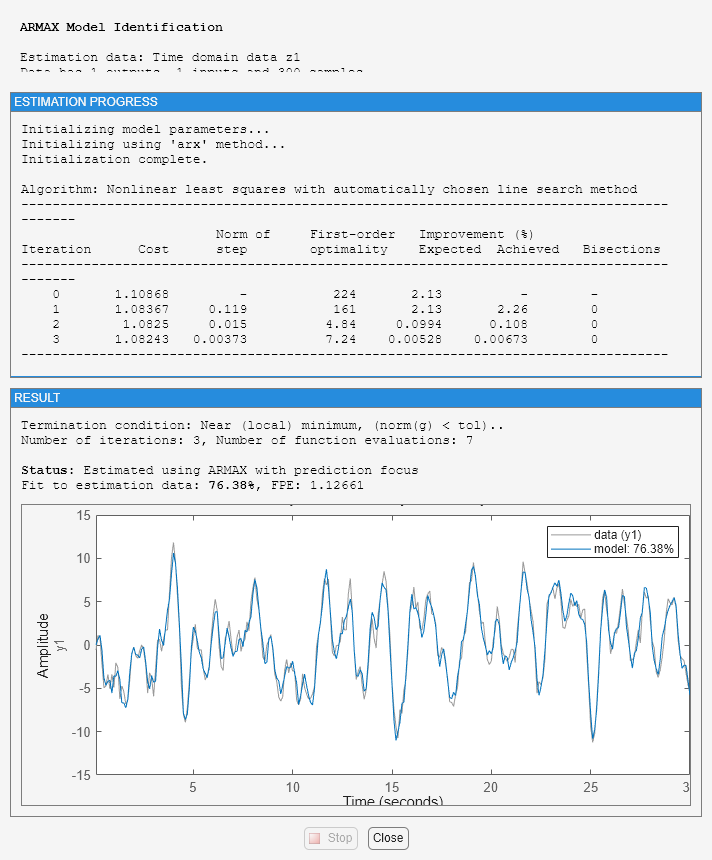Transformations to state-space, transfer function and zeros/poles are obtained by `idssdata`, `tfdata` and `zpkdata`:

```[num,den] = tfdata(m1,'v') ```
```num = 0 0.9430 0.5224 den = 1.0000 -1.5312 0.7293 ```

The 'v' means that num and den are returned as vectors and not as cell arrays. The cell arrays are useful to handle multivariable systems. To also retrieve the 1 standard deviation uncertainties in the values of `num` and `den` use:

```[num, den, ~, dnum, dden] = tfdata(m1,'v') ```
```num = 0 0.9430 0.5224 den = 1.0000 -1.5312 0.7293 dnum = 0 0.0607 0.0782 dden = 0 0.0180 0.0147 ```

### Transforming Identified Models into Numeric LTIs of Control System Toolbox™

The objects also connect directly to the Control System Toolbox™ model objects, like `tf`, `ss`, and `zpk`, and can be converted into these LTI objects, if Control System Toolbox is available. For example, `tf` converts an `idpoly` object into a `tf` object.

```CSTBInstalled = exist('tf','class')==8; if CSTBInstalled % check if Control System Toolbox is installed tfm = tf(m1) % convert IDPOLY model m1 into a TF object end ```
```tfm = From input "u1" to output "y1": 0.943 z^-1 + 0.5224 z^-2 ---------------------------- 1 - 1.531 z^-1 + 0.7293 z^-2 Sample time: 0.1 seconds Discrete-time transfer function. ```

When converting an IDLTI model to the Control Systems Toolbox's LTI models, noise component is not retained. To also include the noise channels as regular inputs of the LTI model, use the 'augmented' flag:

```if CSTBInstalled tfm2 = tf(m1,'augmented') end ```
```tfm2 = From input "u1" to output "y1": 0.943 z^-1 + 0.5224 z^-2 ---------------------------- 1 - 1.531 z^-1 + 0.7293 z^-2 From input "v@y1" to output "y1": 1.051 - 1.113 z^-1 + 0.2069 z^-2 -------------------------------- 1 - 1.531 z^-1 + 0.7293 z^-2 Input groups: Name Channels Measured 1 Noise 2 Sample time: 0.1 seconds Discrete-time transfer function. ```

The noise channel is named `v@y1` in the model `tfm2`.

## SupportGet trial now